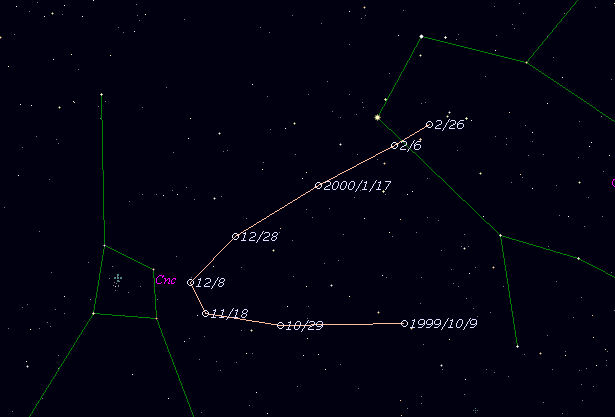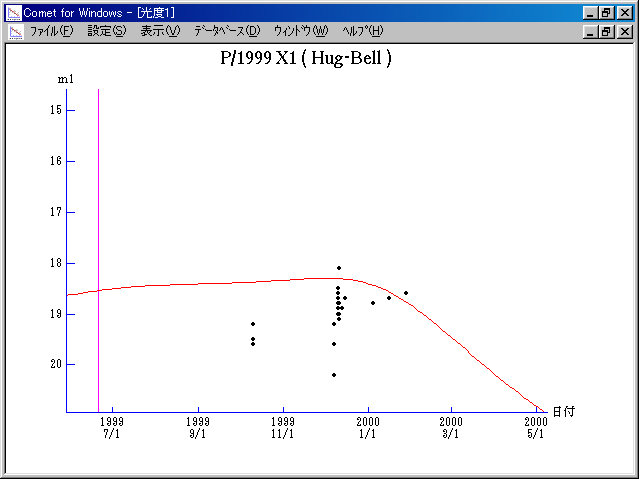# \$B%O%0(B-\$B%Y%kWB@1(B

178P/Hug-Bell (1999)

 English version Home page Updated on July 21, 2006###\$B%W%m%U%#!<%k(B

 \$BId9f(B 178P/1999 X1 \$BH/8+F|(B 1999\$BG/(B12\$B7n(B10\$BF|(B \$BH/8+8wEY(B 18.8\$BEy(B \$BH/8+ Gary Hug, Graham E. Bell (Eskridge, KS)

###\$B50F;MWAG(B

```   The following improved orbital elements by Kenji Muraoka, are
from 88 observations 1999 Oct. 10 to 2000 Feb. 7, perturbations
by 9 Planets, Moon and 5 minor planets were taken into account.
The mean residual is +/- 0.79 arc seconds.

Epoch  =  1999 July  1.0  TT       JDT = 2451360.5
T  =  1999 June 20.83784       +/--0.00256 (m.e.) TT
Peri. =  296.91658                +/- 0.00078
Node  =  103.65119                +/- 0.00075   (2000.0)
Incl. =   10.96890                +/- 0.00043
q  =    1.9367558              +/- 0.0002152 AU
e  =    0.4727865              +/- 0.0000464
a  =    3.6735702              +/- 0.0002495 AU
n  =    0.13998179             +/- 0.00001426
P  =    7.041                  +/- 0.0007174  years
(+/- 0.26 day)
```

###\$B@1?^(B###\$B8wEYJQ2=(B

```        m1 = 13.5 + 5 log\$B&\$(B + 10.0 log r
```##### \$B50F;MWAG\$OB<2,7r<#;a\$N7W;;\$K\$h\$k\$b\$N\$G\$9!#(B \$B@1?^\$O(B StellaNavigator Ver.2.0 for Windows (\$B%"%9%H%m%"!<%D(B \$BJTCx(B / \$B%"%9%-!<=PHG6I4)(B) \$B\$G:n@.\$7\$?\$b\$N\$G\$9!#(B \$B8wEY%0%i%U\$O(BComet for Windows\$B\$G:n@.\$7\$?\$b\$N\$G\$9!#(B